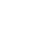# 基于北太真元的仿真建模-二阶电路动态系统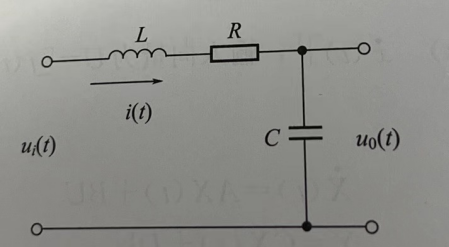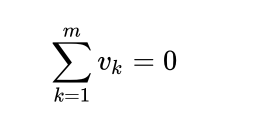L di(t)/dt + 1/C∫i(t)dt + Ri(t) = ui(t)

LC d²u0(t)/dt² + RC du0(t)/dt + u0(t) = ui(t)

d²u0(t)/dt² + 0.5 du0(t)/dt + 0.25u0(t) =  0.25ui(t)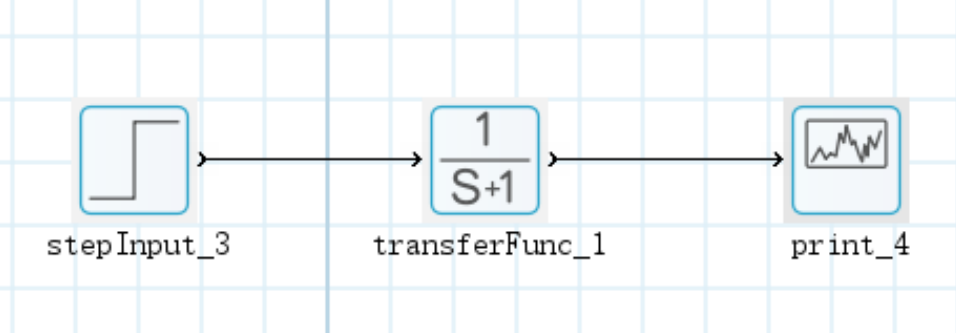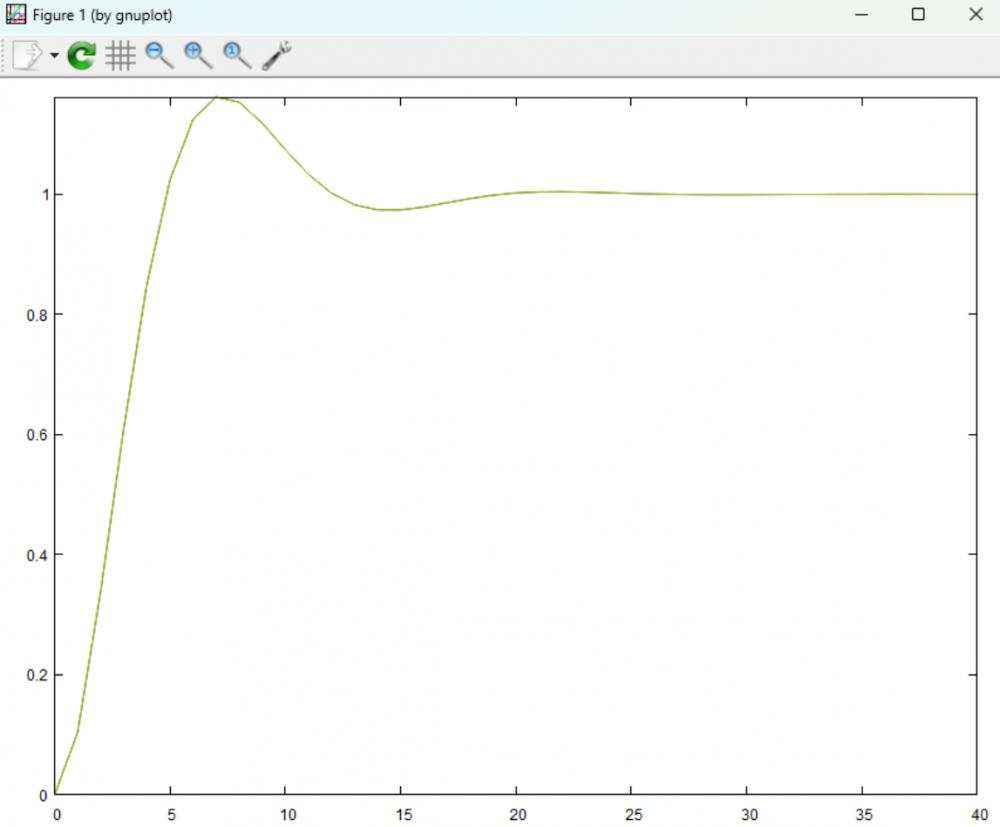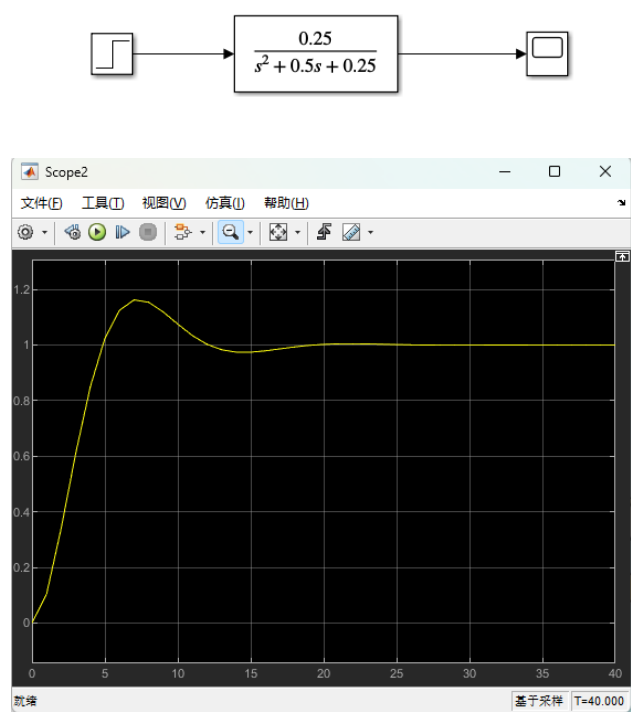280 0 0 收藏 回复

## 回复

### 社区牛人